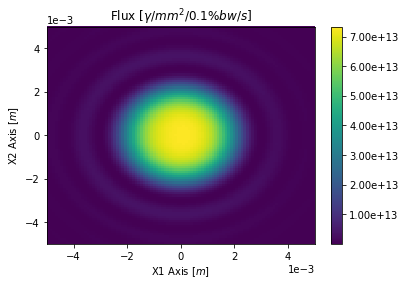# Undulator Flux¶

This is a simple example of how to calculate flux density on an observation plane from an undulator.

In :
# Plots inline for notebook
%matplotlib inline

# Import the OSCARS SR module
import oscars.sr

# Optionally import the plotting tools (matplotlib)
from oscars.plots_mpl import *

OSCARS v2.1.8 - Open Source Code for Advanced Radiation Simulation
Brookhaven National Laboratory, Upton NY, USA
http://oscars.bnl.gov
oscars@bnl.gov

In :
# Create a new OSCARS object.  Default to 8 threads and always use the GPU if available


## Undulator Field¶

Create a simple undulator field

In :
# Clear all existing fields and create an undulator field
osr.clear_bfields()
osr.add_bfield_undulator(bfield=[0, 1, 0], period=[0, 0, 0.050], nperiods=31)


## Particle Beam and Trajectory¶

Define a particle beam, in this case a 3 [GeV] electron beam. You must also define the start and stop times for the calculation.

In :
# Define simple electron beam
osr.set_particle_beam(energy_GeV=3, x0=[0, 0, -1], current=0.5)

# Define the start and stop times for the calculation
osr.set_ctstartstop(0, 2)


## Calculate Flux¶

Calculate the spectrum 30 [m] downstream from the center of the device on a rectangular surface.

In :
# Calculate spectrum at 30 [m].  Note use of the nthreads argument.
flux = osr.calculate_flux_rectangle(
plane='XY',
energy_eV=143.8,
width=[0.01, 0.01],
npoints=[101, 101],
translation=[0, 0, 30]
)


## Plot Flux¶

With the oscars.plots_mpl module you can plot the flux (and many other things)

In :
# Plot flux
plot_flux(flux)